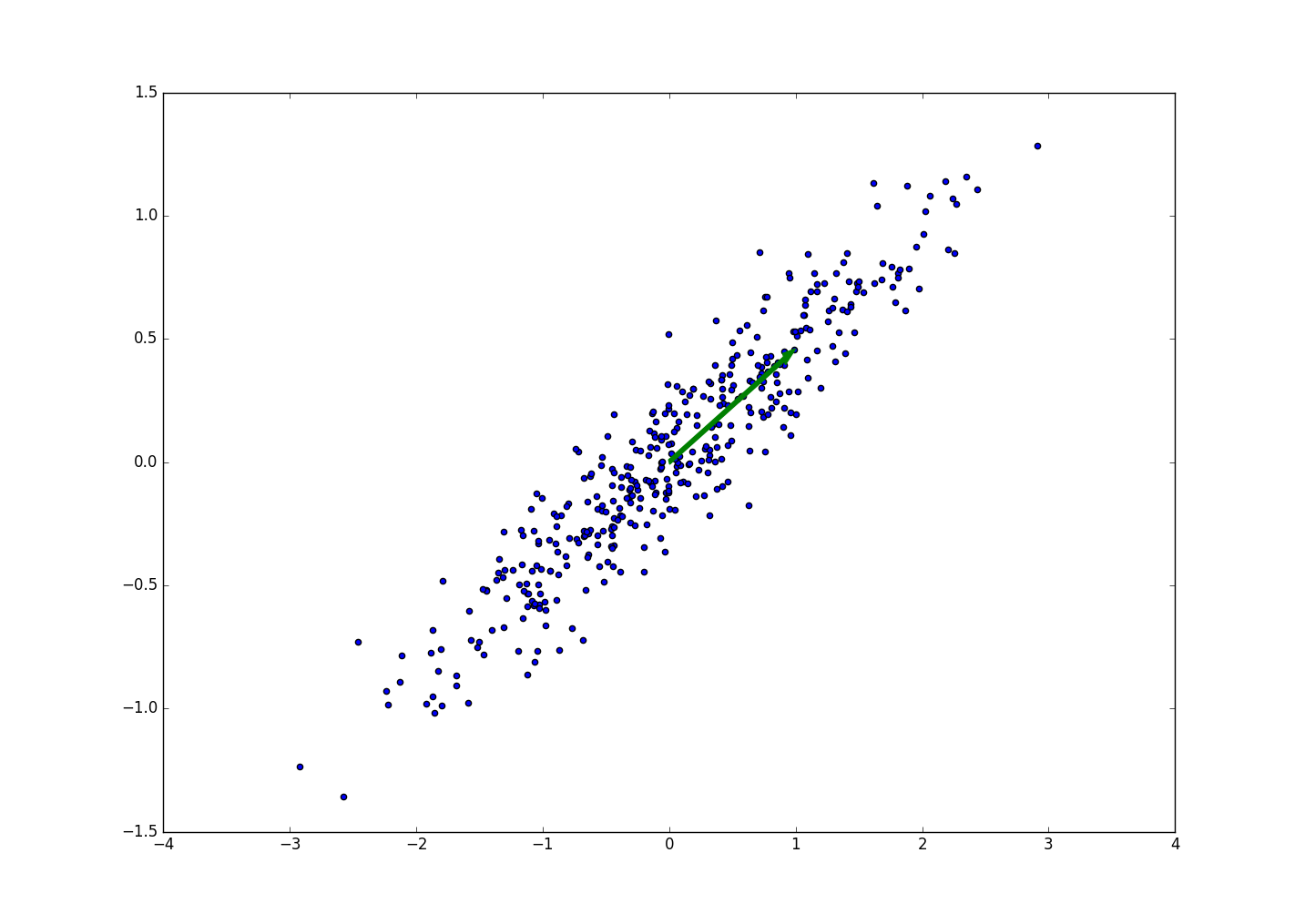0%

### 基于协方差和特征向量的推导$\boldsymbol S=\frac{1}{M}\sum_{}{}(\boldsymbol x_m- \boldsymbol x^-)(\boldsymbol x_m - \boldsymbol x^-)^T$

$\boldsymbol u^T \boldsymbol S \boldsymbol u + \lambda (1 - \boldsymbol u^T \boldsymbol u)$

$\boldsymbol S \boldsymbol u = \lambda \boldsymbol u$

### 对角化协方差矩阵

PCA另一个数学理解角度就是对角化协方差矩阵。我们知道协方差矩阵表示不同特征维度之间的相关性。我们的优化目标：首先利用线性变换，将原始数据变换为一组各维度线性无关的表示，然后在线性无关的各维度中，挑选主要的特征分量，忽略次要的，这就是降维。就是找到低维特征空间中的一组正交基来表示高纬度的特征信息，说白了就是要求映射得到的低维特征之间的线性相关性为0，且各个维度特征之间的方差最大。

PCA降维的步骤总结：

• 将 $X$ 的每一行（表示一个维度的特征）进行零均值化，即减去这一行的均值；
• 求原始数据的协方差矩阵：$C=\frac{1}{m}XX^T$；
• 求协方差矩阵的特征值以及对应的特征向量；
• 将特征向量对应特征值大小从上到下，按行排列，取前 $k$ 行组成变换矩阵 $P$ （大小是 $k \cdot n$ ）；
• $Y=PX$ 即为降维后的矩阵，$Y$ 的大小是 $k \cdot m$。